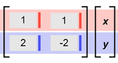# Solving Linear Systems (Matrices and Special Solutions)

### DESCRIPTION

Explore systems of linear equations, and how many solutions a system can have. Express systems in matrix form. See how the determinant of the coefficient matrix reveals how many solutions a system of equations has. Also, use a draggable green point to see what it means for an (x, y) point to be a solution of an equation, or of a system of equations.

Full Lesson Info